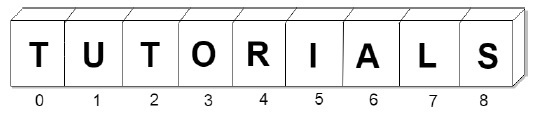# Rust - Slices

A slice is a pointer to a block of memory. Slices can be used to access portions of data stored in contiguous memory blocks. It can be used with data structures like arrays, vectors and strings. Slices use index numbers to access portions of data. The size of a slice is determined at runtime.

Slices are pointers to the actual data. They are passed by reference to functions, which is also known as borrowing.

For example, slices can be used to fetch a portion of a string value. A sliced string is a pointer to the actual string object. Therefore, we need to specify the starting and ending index of a String. Index starts from 0 just like arrays.

### Syntax

```let sliced_value = &data_structure[start_index..end_index]
```

The minimum index value is 0 and the maximum index value is the size of the data structure. NOTE that the end_index will not be included in final string.

The diagram below shows a sample string Tutorials, that has 9 characters. The index of the first character is 0 and that of the last character is 8.The following code fetches 5 characters from the string (starting from index 4).

```fn main() {
let n1 = "Tutorials".to_string();
println!("length of string is {}",n1.len());
let c1 = &n1[4..9];

// fetches characters at 4,5,6,7, and 8 indexes
println!("{}",c1);
}
```

### Output

```length of string is 9
rials
```

### Illustration - Slicing an integer array

The main() function declares an array with 5 elements. It invokes the use_slice() function and passes to it a slice of three elements (points to the data array). The slices are passed by reference. The use_slice() function prints the value of the slice and its length.

```fn main(){
let data = [10,20,30,40,50];
use_slice(&data[1..4]);
//this is effectively borrowing elements for a while
}
fn use_slice(slice:&[i32]) {
// is taking a slice or borrowing a part of an array of i32s
println!("length of slice is {:?}",slice.len());
println!("{:?}",slice);
}
```

### Output

```length of slice is 3
[20, 30, 40]
```

## Mutable Slices

The &mut keyword can be used to mark a slice as mutable.

```fn main(){
let mut data = [10,20,30,40,50];
use_slice(&mut data[1..4]);
// passes references of
20, 30 and 40
println!("{:?}",data);
}
fn use_slice(slice:&mut [i32]) {
println!("length of slice is {:?}",slice.len());
println!("{:?}",slice);
slice = 1010; // replaces 20 with 1010
}
```

### Output

```length of slice is 3
[20, 30, 40]
[10, 1010, 30, 40, 50]
```

The above code passes a mutable slice to the use_slice() function. The function modifies the second element of the original array.